1-to-1 Functions

1-to-1 Functions

A 1-to-1 function passes a vertical line test and a horizontal line test.

This record is not due to good test-taking skills, but it is due to the function's domain and range. Every x-value of a 1:1 function can yield only one possible value of y (as with any function), and every y-value corresponds with only one value of x.

Which of the following graphs shows a 1:1 function?

y2 = x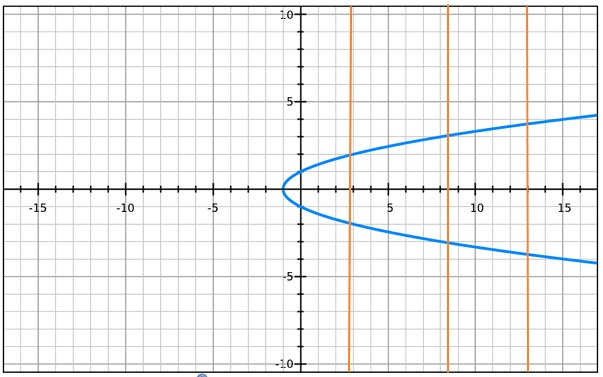This equation does not pass the vertical line test, so it is not a function. On this graph, most values of x have more than one possible value of y.

y = x2 +1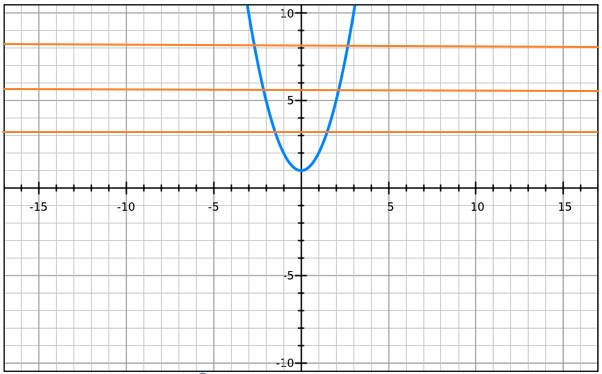This equation is a function, but it does not pass the horizontal line test. On this graph, most values of y have more than one possible value of x.

y = 2x + 1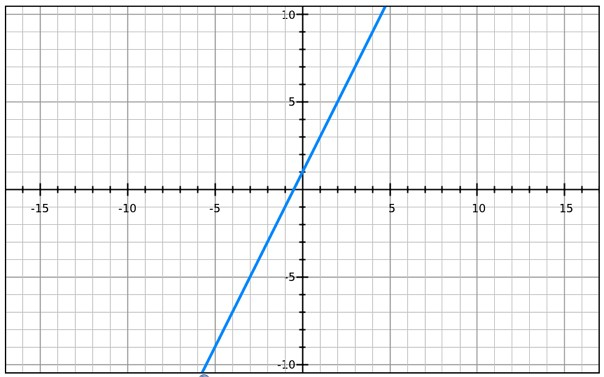The line above represents a 1-to-1 function because each value of x can only yield a single value of y, and vice versa.

But what if you don't have a graphing calculator? How can you determine if a function is a 1-to-1 function?!

Mathematicians have come up with a test:

If f(p) = f(q), then p = q.  If this fact holds, then congratulations! You have a 1-to-1 function.

If our function is f(x) = 2x + 1, then

f(p) = f(q)

2(p) + 1 = 2(q) + 1

2(p) + 1 - 1 = 2(q) + 1 - 1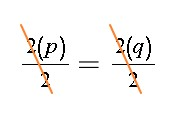So, p = q.

Let's see what happens when we try it with one of the other equations:

If our function is f(x) = (2x2 + 1), then

f(p) = f(q)

(p2 + 1) = (q2 + 1)

(p2 + 1 - 1) = (q2 + 1 - 1)

p2 = q2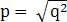The answer to a square root can either be positive or negative, so p and q are not necessarily equal.